How Cheenta works to ensure student success?
Explore the Back-Story

# Math Kangaroo (Benjamin) 2014 Problem No 24Try this beautiful Problem based on simple Algebra appeared in Math Kangaroo (Benjamin) 2014 Problem 24.

## Math Kangaroo (Benjamin) 2014 | Problem No 24

Grandma gives 180 marbles to her ten grandchildren. No two children get the same amount of marbles. Anna gets the most. What is the minimum number of marbles that Anna could get?

• 19
• 20
• 21
• 22
• 23

Arithmetic

Equation solving

Algebra

## Suggested Book | Source | Answer

Algebra by Gelfand

Math Kangaroo (Benjamin), 2014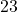## Try with Hints

Here

Number of children is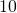.
Number of marbles is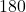.
And Anna gets the most and no 2 children gets the same number of marbles.

Let us assume that Anna could get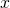marbles and also the other 9 children receiving 1 less each step. Apply the condition to construct the equation

The equation will be-+-+-+-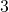+-+-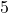+-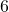+-+-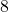+-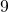=Now solve for.

So, Anna can havemarbles.

## Subscribe to Cheenta at Youtube

Try this beautiful Problem based on simple Algebra appeared in Math Kangaroo (Benjamin) 2014 Problem 24.

## Math Kangaroo (Benjamin) 2014 | Problem No 24

Grandma gives 180 marbles to her ten grandchildren. No two children get the same amount of marbles. Anna gets the most. What is the minimum number of marbles that Anna could get?

• 19
• 20
• 21
• 22
• 23

Arithmetic

Equation solving

Algebra

## Suggested Book | Source | Answer

Algebra by Gelfand

Math Kangaroo (Benjamin), 2014## Try with Hints

Here

Number of children is.
Number of marbles is.
And Anna gets the most and no 2 children gets the same number of marbles.

Let us assume that Anna could getmarbles and also the other 9 children receiving 1 less each step. Apply the condition to construct the equation

The equation will be-+-+-+-+-+-+-+-+-+-=Now solve for.

So, Anna can havemarbles.

## Subscribe to Cheenta at Youtube

This site uses Akismet to reduce spam. Learn how your comment data is processed.

### Knowledge Partner